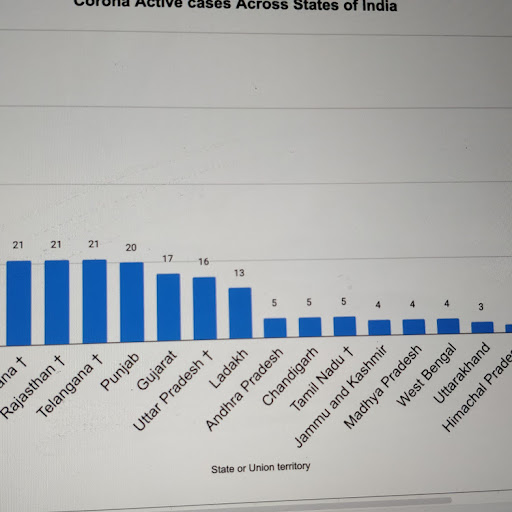## Blogadmin
Mar 8 th, 2016

## Aptitude Grasp Patron – 2(pic source: www.mathinenglish.com)
It’s by simple addition so in
1st pic it’s 16+17 = 33
2nd pic 8*8= 64(pic source: www.learn-with-math-games.com)
6*2 = 12*3 = 36*4 = 144
5*3 = 15*4 = 60*5 = 300
12*3 = 36*4 = 144*5 = 720(pic source: www.indiabix.com)
Vertically adding all numbers will give 14 i.e.
7+2+1+4 = 14
3+8+1+2 = 14
6+5+2+1 = 14
2+4+4+4 = 14
So, Answer is 4.(pic source: puzzlersworld.com)
1+2+3 = 1+2+3 = 6 i.e. 6*1
Similarly, 2+4+6 = 2+4+6 = 12 i.e. 6*2
3+6+9 = 3+6+9 = 18 i.e. 6*3(pic source: magicalrosebud.wordpress.com)
Very easy, each number is equal to sum of two numbers adjacent to it’s opposite number i.e. ? = 9+8 = 17(pic source: 24x7coaching.com)
5*4*6 = 120
5*6*3 = 90
3*2*7 = 42(pic source: puzzlersworld.com)
Sum of opposite numbers is 15
i.e. 12+3 = 6+9 = 8+7 = 5+7 = 15